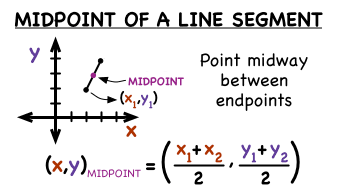# What is the midpoint of a segment whose endpoints are (4, -2) and (5, 1)?

May 28, 2018

$\left(\frac{9}{2} , \frac{- 1}{2}\right)$

#### Explanation:

The midpoint formula is shown here:We are given the two endpoints, so we can plug it into the formula to find the midpoint. Notice that the formula is same as the average of the two x-values and y-values.

$\text{Midpoint} = \left(\frac{4 + 5}{2} , \frac{- 2 + 1}{2}\right)$

$\quad \quad \quad \quad \quad \quad \quad \quad = \left(\frac{9}{2} , \frac{- 1}{2}\right)$

Hope this helps!